2021 NFL MVP Odds
+450
4.5 to 1Dak Prescott
13.4% implied probability

+450
4.5 to 1Kyler Murray
13.4% implied probability

+800
8 to 1Tom Brady
8.2% implied probability

+1200
12 to 1Matthew Stafford
5.7% implied probability

+1200
12 to 1Aaron Rodgers
5.7% implied probability

+1400
14 to 1Justin Herbert
4.9% implied probability

+1800
18 to 1Patrick Mahomes
3.9% implied probability

+2000
20 to 1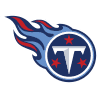Derrick Henry
3.5% implied probability

+5000
50 to 1Teddy Bridgewater
1.4% implied probability

+5000
50 to 1Kirk Cousins
1.4% implied probability

+6500
65 to 1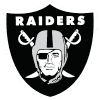Derek Carr
1.1% implied probability

+6500
65 to 1Davante Adams
1.1% implied probability

+10000
100 to 1Alvin Kamara
0.7% implied probability

+10000
100 to 1Cooper Kupp
0.7% implied probability

+10000
100 to 1Sam Darnold
0.7% implied probability

+10000
100 to 1CeeDee Lamb
0.7% implied probability

+10000
100 to 1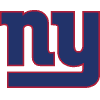Saquon Barkley
0.7% implied probability

+10000
100 to 1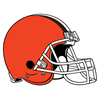Baker Mayfield
0.7% implied probability

+10000
100 to 1Deebo Samuel
0.7% implied probability

+10000
100 to 1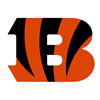Joe Burrow
0.7% implied probability

+10000
100 to 1Aaron Donald
0.7% implied probability

+10000
100 to 1Amari Cooper
0.7% implied probability

+10000
100 to 1Taylor Heinicke
0.7% implied probability

+10000
100 to 1Jalen Hurts
0.7% implied probability

+10000
100 to 1Nick Chubb
0.7% implied probability

+10000
100 to 1Ezekiel Elliott
0.7% implied probability

+10000
100 to 1Jimmy Garoppolo
0.7% implied probability

+10000
100 to 1Ryan Tannehill
0.7% implied probability

+10000
100 to 1Jameis Winston
0.7% implied probability

+10000
100 to 1DeAndre Hopkins
0.7% implied probability

+15000
150 to 1James Robinson
0.5% implied probability

+15000
150 to 1Terry McLaurin
0.5% implied probability

+15000
150 to 1Courtland Sutton
0.5% implied probability

+15000
150 to 1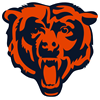Tarik Cohen
0.5% implied probability

+15000
150 to 1Kenyan Drake
0.5% implied probability

+15000
150 to 1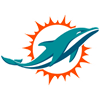Will Fuller
0.5% implied probability

+15000
150 to 1Julio Jones
0.5% implied probability

+15000
150 to 1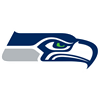Tyler Lockett
0.5% implied probability

+15000
150 to 1Justin Jefferson
0.5% implied probability

+15000
150 to 1Mike Evans
0.5% implied probability

+15000
150 to 1Calvin Ridley
0.5% implied probability

+15000
150 to 1Clyde Edwards-Helaire
0.5% implied probability

+15000
150 to 1Allen Robinson
0.5% implied probability

+15000
150 to 1George Kittle
0.5% implied probability

+15000
150 to 1Tyreek Hill
0.5% implied probability

+20000
200 to 1Aaron Jones
0.4% implied probability

+20000
200 to 1Todd Gurley
0.4% implied probability

+20000
200 to 1Stefon Diggs
0.4% implied probability

+20000
200 to 1Joe Mixon
0.4% implied probability

+20000
200 to 1Jacoby Brissett
0.4% implied probability

+20000
200 to 1Dalvin Cook
0.4% implied probability

+20000
200 to 1Mitchell Trubisky
0.4% implied probability

+20000
200 to 1Chris Godwin
0.4% implied probability

+20000
200 to 1Kareem Hunt
0.4% implied probability

+20000
200 to 1Austin Ekeler
0.4% implied probability

+20000
200 to 1Chris Carson
0.4% implied probability

+20000
200 to 1Leonard Fournette
0.4% implied probability

+20000
200 to 1Matt Ryan
0.4% implied probability

+20000
200 to 1Myles Gaskin
0.4% implied probability

+20000
200 to 1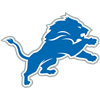D'Andre Swift
0.4% implied probability

+20000
200 to 1Ryan Fitzpatrick
0.4% implied probability

+20000
200 to 1Antonio Gibson
0.4% implied probability

+20000
200 to 1Jared Goff
0.4% implied probability

+20000
200 to 1Adam Thielen
0.4% implied probability

+20000
200 to 1Le'Veon Bell
0.4% implied probability

+20000
200 to 1Antonio Brown
0.4% implied probability

+20000
200 to 1Keenan Allen
0.4% implied probability

+20000
200 to 1Odell Beckham
0.4% implied probability

+20000
200 to 1Ben Roethlisberger
0.4% implied probability

+20000
200 to 1Daniel Jones
0.4% implied probability

+20000
200 to 1Miles Sanders
0.4% implied probability

+20000
200 to 1David Montgomery
0.4% implied probability

+20000
200 to 1Josh Jacobs
0.4% implied probability

+20000
200 to 1Taysom Hill
0.4% implied probability

+20000
200 to 1Drew Lock
0.4% implied probability

+20000
200 to 1Travis Kelce
0.4% implied probability

+20000
200 to 1Jonathan Taylor
0.4% implied probability

+20000
200 to 1Tua Tagovailoa
0.4% implied probability

+20000
200 to 1Melvin Gordon
0.4% implied probability

+20000
200 to 1Carson Wentz
0.4% implied probability

+20000
200 to 1Trey Lance
0.4% implied probability

+20000
200 to 1Zach Wilson
0.4% implied probability

+20000
200 to 1Mac Jones
0.4% implied probability

+20000
200 to 1Justin Fields
0.4% implied probability

+20000
200 to 1A.J. Brown
0.4% implied probability

+25000
250 to 1Christian McCaffrey
0.3% implied probability

+25000
250 to 1Russell Wilson
0.3% implied probability

+50000
500 to 1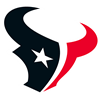Tyrod Taylor
0.1% implied probability

+50000
500 to 1Raheem Mostert
0.1% implied probability

+50000
500 to 1Deshaun Watson
0.1% implied probability

+50000
500 to 1Trevor Lawrence
0.1% implied probability